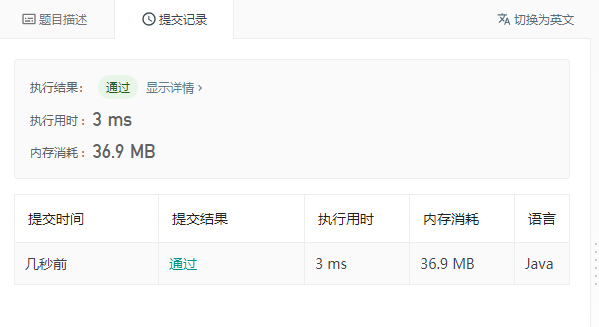`输入: amount = 5, coins = [1, 2, 5]输出: 4解释: 有四种方式可以凑成总金额:5=55=2+2+15=2+1+1+15=1+1+1+1+1`

`输入: amount = 3, coins = 输出: 0解释: 只用面额2的硬币不能凑成总金额3。`

`输入: amount = 10, coins =  输出: 1`

• 0 <= amount (总金额) <= 5000
• 1 <= coin (硬币面额) <= 5000
• 硬币种类不超过 500 种
• 结果符合 32 位符号整数

class Solution {

public int change(int amount, int[] coins) {

if(amount==0) return 1;

if(coins.length == 0) return 0;

int[] dp = new int[amount+1];

dp = 1;

for(int i = 0; i < coins.length; i++){

for(int j=coins[i]; j<= amount;j++){

dp[j] += dp[j-coins[i]];

}

}

return dp[amount];

}

}#### 最新留言

[***.36.149.33]2022年07月29日 14时23分56秒

## 关于作者喝酒易醉，品茶养心，人生如梦，品茶悟道，何以解忧？唯有杜康！
-- 愿君每日到此一游！